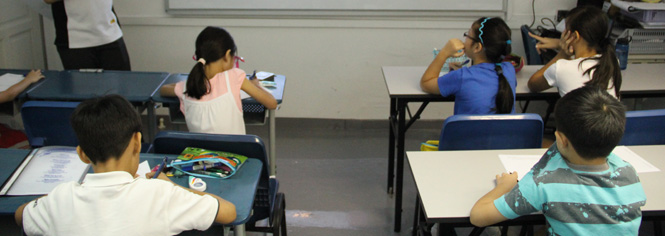# Fusion Math S1 (13 yr)Objectives

1. Generate interest in Mathematics and equip students with Mathematical knowledge.
2. Enhance students' problem solving skills and strengthen their abilities to attempt challenging Mathematical problems similar to SMO (Junior).
3. Head start for all Primary 6 students (after their PSLE) to learn Secondary IP Math syllabus (Module 1 & 2) in advance through acquiring algebra and analytical geometry knowledge.
4. Primary 4 and 5 students (selective) who are keen to learn in advance can participate.

 Semester 1(Jan - Mar) - Revision on Algebraic   Topics - Word Problems   (%, Rate & Speed)- Logic Problems- Completing the Squares- Sum & Product of Roots- Divisibility- Number Theory- Congruence Semester 1 (Mar - May)- Indicies & Surds- Algebraic Identities- Inequalities (AM-GM)- Geometry (Angles)- Geometry (Lengths)- Geometry (Areas)- Permutation &   Combinations- Combinatorics   (Counting)- Revision Papers Semester 2(Jun - Sep) - AMC Practice Papers- Number Patterns- Series (Summation)- Pythagoras' Theorem- Triangles- Quadrilaterals- Circles Properties Semester 2(Sep - Nov) - Sets- Binomial Theorem- Algebraic Identities- Linear Inequalities- Quadratic Inequalities- Discriminant &   Nature of Roots- Number Theory   (Factorization)

 Module 1Semester 2 (Oct - Nov) / Year End Holiday - Real Numbers- Approximation & Estimation, HCF & LCM- Basic Algebra- Algebra Expansion- Algebra Factorisation - Linear Equation- Linear Graph- Pythagoras’ Theorem- Probability Module 2Semester 2  (Nov) / Year End Holiday - Quadratic Equations- Algebraic Manipulation I- Algebraic Manipulation II- Simultaneous Equations - Indices - Surds- Modulus- Quadratic Graph- Congruent & Similar Triangles

Topics covered are subjected to change depending on the ability of students.